Students can download 5th Maths Term 1 Chapter 2 Numbers Ex 2.9 Questions and Answers, Notes, Samacheer Kalvi 5th Maths Guide Pdf helps you to revise the complete Tamilnadu State Board New Syllabus, helps students complete homework assignments and to score high marks in board exams.

## Tamilnadu Samacheer Kalvi 5th Maths Solutions Term 1 Chapter 2 Numbers Ex 2.9

Question 1.
a. A cement factory produces 37500 bags of cements in a month (30 days). How many cement bags were produced in one day?
Total number of bags of cement produced by factor per month = 37500
∴ No of bag of cement produced per day
= 37500 ÷ 30
= 1,250 bags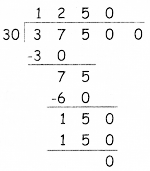b. 8075 mangoes were harvested from a mango garden. 95 mangoes are packed in each package. How many packages will be there?
Total number of mangoes = 8075
Number of mangos in one packet = 95
∴ Total number of packages = 8075 ÷ 95
= 85 packages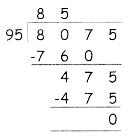c. 25 families living in a street needed 1625 liters of water per day. How much of water is needed by one family?
Total No. of families in a street = 25
Total litres of water needed = 1625
∴ Water is needed by one family = 1625 ÷ 25
= 65 litres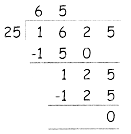d. 6750 bananas have to be loaded in a tempo van. If 15 bananas are arranged in one basket, then how many baskets will be needed to arrange
all the bananas?
Total Number of bananas = 6750
Arranged Equally in a basket = 15
∴ Number of bananas in one basket = 6750 ÷ 15
= 450Question 2.
Divide the following.
a. 4525 ÷ 15
b. 3448 ÷ 24
c. 7342 ÷ 18
d. 3626 ÷ 37
e. 4872 ÷56
a. 4525 ÷ 15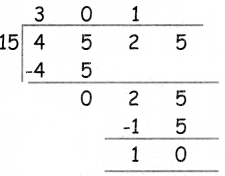b. 3448 ÷ 24c. 7342 ÷ 18d. 3626 ÷ 37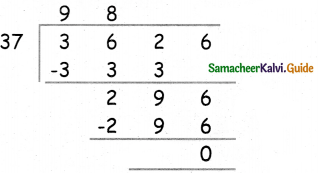e. 4872 ÷56